# Fraction calculator

The calculator performs basic and advanced operations with fractions, expressions with fractions combined with integers, decimals, and mixed numbers. It also shows detailed step-by-step information about the fraction calculation procedure. Solve problems with two, three, or more fractions and numbers in one expression.

## Result:

### 41/8 + 21/16 = 99/16 = 6 3/16 = 6.1875

Spelled result in words is ninety-nine sixteenths (or six and three sixteenths).

### How do you solve fractions step by step?

1. Conversion a mixed number 4 1/8 to a improper fraction: 4 1/8 = 4 1/8 = 4 · 8 + 1/8 = 32 + 1/8 = 33/8

To find a new numerator:
a) Multiply the whole number 4 by the denominator 8. Whole number 4 equally 4 * 8/8 = 32/8
b) Add the answer from previous step 32 to the numerator 1. New numerator is 32 + 1 = 33
c) Write a previous answer (new numerator 33) over the denominator 8.

Four and one eighth is thirty-three eighths
2. Conversion a mixed number 2 1/16 to a improper fraction: 2 1/16 = 2 1/16 = 2 · 16 + 1/16 = 32 + 1/16 = 33/16

To find a new numerator:
a) Multiply the whole number 2 by the denominator 16. Whole number 2 equally 2 * 16/16 = 32/16
b) Add the answer from previous step 32 to the numerator 1. New numerator is 32 + 1 = 33
c) Write a previous answer (new numerator 33) over the denominator 16.

Two and one sixteenth is thirty-three sixteenths
3. Add: 33/8 + 33/16 = 33 · 2/8 · 2 + 33/16 = 66/16 + 33/16 = 66 + 33/16 = 99/16
For adding, subtracting, and comparing fractions, it is suitable to adjust both fractions to a common (equal, identical) denominator. The common denominator you can calculate as the least common multiple of both denominators - LCM(8, 16) = 16. In practice, it is enough to find the common denominator (not necessarily the lowest) by multiplying the denominators: 8 × 16 = 128. In the following intermediate step, the fraction result cannot be further simplified by canceling.
In other words - thirty-three eighths plus thirty-three sixteenths = ninety-nine sixteenths.

#### Rules for expressions with fractions:

Fractions - use the slash “/” between the numerator and denominator, i.e., for five-hundredths, enter 5/100. If you are using mixed numbers, be sure to leave a single space between the whole and fraction part.
The slash separates the numerator (number above a fraction line) and denominator (number below).

Mixed numerals (mixed fractions or mixed numbers) write as non-zero integer separated by one space and fraction i.e., 1 2/3 (having the same sign). An example of a negative mixed fraction: -5 1/2.
Because slash is both signs for fraction line and division, we recommended use colon (:) as the operator of division fractions i.e., 1/2 : 3.

Decimals (decimal numbers) enter with a decimal point . and they are automatically converted to fractions - i.e. 1.45.

The colon : and slash / is the symbol of division. Can be used to divide mixed numbers 1 2/3 : 4 3/8 or can be used for write complex fractions i.e. 1/2 : 1/3.
An asterisk * or × is the symbol for multiplication.
Plus + is addition, minus sign - is subtraction and ()[] is mathematical parentheses.
The exponentiation/power symbol is ^ - for example: (7/8-4/5)^2 = (7/8-4/5)2

#### Examples:

subtracting fractions: 2/3 - 1/2
multiplying fractions: 7/8 * 3/9
dividing Fractions: 1/2 : 3/4
exponentiation of fraction: 3/5^3
fractional exponents: 16 ^ 1/2
adding fractions and mixed numbers: 8/5 + 6 2/7
dividing integer and fraction: 5 ÷ 1/2
complex fractions: 5/8 : 2 2/3
decimal to fraction: 0.625
Fraction to Decimal: 1/4
Fraction to Percent: 1/8 %
comparing fractions: 1/4 2/3
multiplying a fraction by a whole number: 6 * 3/4
square root of a fraction: sqrt(1/16)
reducing or simplifying the fraction (simplification) - dividing the numerator and denominator of a fraction by the same non-zero number - equivalent fraction: 4/22
expression with brackets: 1/3 * (1/2 - 3 3/8)
compound fraction: 3/4 of 5/7
fractions multiple: 2/3 of 3/5
divide to find the quotient: 3/5 ÷ 2/3

The calculator follows well-known rules for order of operations. The most common mnemonics for remembering this order of operations are:
PEMDAS - Parentheses, Exponents, Multiplication, Division, Addition, Subtraction.
BEDMAS - Brackets, Exponents, Division, Multiplication, Addition, Subtraction
BODMAS - Brackets, Of or Order, Division, Multiplication, Addition, Subtraction.
GEMDAS - Grouping Symbols - brackets (){}, Exponents, Multiplication, Division, Addition, Subtraction.
Be careful, always do multiplication and division before addition and subtraction. Some operators (+ and -) and (* and /) has the same priority and then must evaluate from left to right.

## Fractions in word problems:

• A dumpA dump truck bought 1/3 of a ton of rock on the first trip, 1/2 of a ton on the second trip, and 4/5 of a ton on the third trip. What was the total weight of the rock?
• Team runThe first team member in a 926-person relay race must run 2 1/4 laps, the second team member must run 1 1/2 laps, and the third team member must run 3 1/4 laps. How many laps in all must each team run?
• Sum of 18Sum of two fractions is 4 3/7. If one of the fractions is 2 1/5 find the other one .
• The petAnanya has a bunny. She bought 4 7/8 pounds of carrots. She fed her bunny 1 1/4 pounds of carrots the first week. She fed her bunny 5/6 pounds of carrots the second week. All together, how many pounds of carrots did she feed her bunny? 1. Draw a tape diag
• Tartlets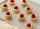After a special event, a caterer examined the leftovers on the serving table. She saw 10/11 of a tartlet with apples, 2/3 of a tartlet with strawberries, and 10/11 of a tartlet with raspberries. How many leftover tartlets did the caterer have?
• Jo walks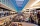Jo walks 3/4 of km to a friends home, 1/2 km to mall, and 2/3 km home. What total distance that joy covers?
• Calculate 20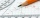Calculate the sum of 1/5 of a right angle and 3/4 of a right angle and 3/4 of a straight angleWhy does 1 3/4 + 2 9/10 equal 4.65? How do you solve this?It took Jose two-thirds of an hour to complete his math homework on Monday, three-fourths of an hour on Tuesday, any two- fifths of an hour on Wednesday. How many hours did it take Jose to complete his homework altogether?What is 4 1/2+2/7-213/14?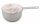Monica’s cookie recipe calls for Three-fourths of a cup of flour. Her mother’s recipe calls for Two-thirds as much as Monica’s. How many cups of flour does her mother’s recipe require?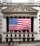For the week of July 22, the following day to day changes in the stock market was recorded a certain stock: -2 on Monday; +4 Tuesday; -8 Wednesday; + 2 1/2 Thursday; - 3 1/4 Friday. The stock began the week at 78 points. How many points did it finish withSkilled workshop master washes client car 1/5 hour, cleaned the client's car in 5/4 hour, and painted small defects on car 1 1/3 hour. How long did it take him to do all the necessary work tasks?